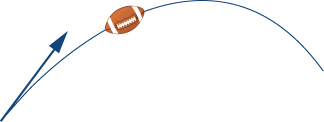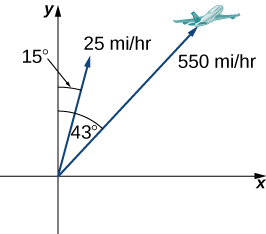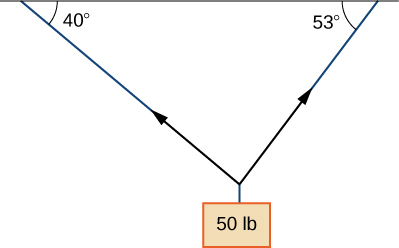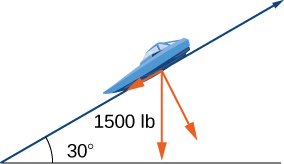$$\newcommand{\id}{\mathrm{id}}$$ $$\newcommand{\Span}{\mathrm{span}}$$ $$\newcommand{\kernel}{\mathrm{null}\,}$$ $$\newcommand{\range}{\mathrm{range}\,}$$ $$\newcommand{\RealPart}{\mathrm{Re}}$$ $$\newcommand{\ImaginaryPart}{\mathrm{Im}}$$ $$\newcommand{\Argument}{\mathrm{Arg}}$$ $$\newcommand{\norm}{\| #1 \|}$$ $$\newcommand{\inner}{\langle #1, #2 \rangle}$$ $$\newcommand{\Span}{\mathrm{span}}$$

# Exercises for Vectors in the Plane

$$\newcommand{\vecs}{\overset { \rightharpoonup} {\mathbf{#1}} }$$ $$\newcommand{\vecd}{\overset{-\!-\!\rightharpoonup}{\vphantom{a}\smash {#1}}}$$$$\newcommand{\id}{\mathrm{id}}$$ $$\newcommand{\Span}{\mathrm{span}}$$ $$\newcommand{\kernel}{\mathrm{null}\,}$$ $$\newcommand{\range}{\mathrm{range}\,}$$ $$\newcommand{\RealPart}{\mathrm{Re}}$$ $$\newcommand{\ImaginaryPart}{\mathrm{Im}}$$ $$\newcommand{\Argument}{\mathrm{Arg}}$$ $$\newcommand{\norm}{\| #1 \|}$$ $$\newcommand{\inner}{\langle #1, #2 \rangle}$$ $$\newcommand{\Span}{\mathrm{span}}$$ $$\newcommand{\id}{\mathrm{id}}$$ $$\newcommand{\Span}{\mathrm{span}}$$ $$\newcommand{\kernel}{\mathrm{null}\,}$$ $$\newcommand{\range}{\mathrm{range}\,}$$ $$\newcommand{\RealPart}{\mathrm{Re}}$$ $$\newcommand{\ImaginaryPart}{\mathrm{Im}}$$ $$\newcommand{\Argument}{\mathrm{Arg}}$$ $$\newcommand{\norm}{\| #1 \|}$$ $$\newcommand{\inner}{\langle #1, #2 \rangle}$$ $$\newcommand{\Span}{\mathrm{span}}$$

For exercises 1 - 10, consider points $$P(−1,3), Q(1,5),$$ and $$R(−3,7)$$. Determine the requested vectors and express each of them

$$a.$$ in component form and

$$b.$$ by using standard unit vectors.

1) $$\vecd{PQ}$$

a. $$\vecd{PQ}=⟨2,2⟩$$
b. $$\vecd{PQ}=2\hat{\mathbf i}+2\hat{\mathbf j}$$

2) $$\vecd{PR}$$

3) $$\vecd{QP}$$

a. $$\vecd{QP}=⟨−2,−2⟩$$
b. $$\vecd{QP}=−2\hat{\mathbf i}−2\hat{\mathbf j}$$

4) $$\vecd{RP}$$

5) $$\vecd{PQ}+\vecd{PR}$$

a. $$\vecd{PQ}+\vecd{PR}=⟨0,6⟩$$
b. $$\vecd{PQ}+\vecd{PR}=6\hat{\mathbf j}$$

6) $$\vecd{PQ}−\vecd{PR}$$

7) $$2\vecd{PQ}−2\vecd{PR}$$

a. $$2\vecd{PQ}→−2\vecd{PR}=⟨8,−4⟩$$
b. $$2\vecd{PQ}−2\vecd{PR}=8\hat{\mathbf i}−4\hat{\mathbf j}$$

8) $$2\vecd{PQ}+\frac{1}{2}\vecd{PR}$$

9) The unit vector in the direction of $$\vecd{PQ}$$

a. $$\left\langle\frac{\sqrt{2}}{2},\frac{\sqrt{2}}{2}\right\rangle$$
b. $$\frac{\sqrt{2}}{2}\hat{\mathbf i}+\frac{\sqrt{2}}{2}\hat{\mathbf j}$$

10) The unit vector in the direction of $$\vecd{PR}$$

11) A vector $${\overset{\scriptstyle\rightharpoonup}{\mathbf v}}$$ has initial point $$(−1,−3)$$ and terminal point $$(2,1)$$. Find the unit vector in the direction of $$\vecs v$$. Express the answer in component form.

$$⟨\frac{3}{5},\frac{4}{5}⟩$$

12) A vector $$\vecs v$$ has initial point $$(−2,5)$$ and terminal point $$(3,−1)$$. Find the unit vector in the direction of $$\vecs v$$. Express the answer in component form.

13) The vector $$\vecs v$$ has initial point $$P(1,0)$$ and terminal point $$Q$$ that is on the $$y$$-axis and above the initial point. Find the coordinates of terminal point $$Q$$ such that the magnitude of the vector $$\vecs v$$ is $$\sqrt{5}$$.

$$Q(0,2)$$

14) The vector $$\vecs v$$ has initial point $$P(1,1)$$ and terminal point $$Q$$ that is on the $$x$$-axis and left of the initial point. Find the coordinates of terminal point $$Q$$ such that the magnitude of the vector $$\vecs v$$ is $$\sqrt{10}$$.

For exercises 15 and 16, use the given vectors $$\vecs a$$ and $$\vecs b$$.

a. Determine the vector sum $$\vecs a+\vecs b$$ and express it in both the component form and by using the standard unit vectors.

b. Find the vector difference $$\vecs a −\vecs b$$ and express it in both the component form and by using the standard unit vectors.

c. Verify that the vectors $$\vecs a, \, \vecs b,$$ and $$\vecs a+\vecs b$$, and, respectively, $$\vecs a, \, \vecs b$$, and $$\vecs a−\vecs b$$ satisfy the triangle inequality.

d. Determine the vectors $$2\vecs a, −\vecs b,$$ and $$2\vecs a−\vecs b.$$ Express the vectors in both the component form and by using standard unit vectors.

15) $$\vecs a=2\hat{\mathbf i}+\hat{\mathbf j}, \vecs b=\hat{\mathbf i}+3\hat{\mathbf j}$$

$$a.\, \vecs a+\vecs b=⟨3,4⟩, \quad \vecs a+\vecs b=3\hat{\mathbf i}+4\hat{\mathbf j}$$
$$b.\, \vecs a−\vecs b=⟨1,−2⟩, \quad \vecs a−\vecs b=\hat{\mathbf i}−2\hat{\mathbf j}$$
$$c.$$ Answers will vary
$$d.\, 2\vecs a=⟨4,2⟩, \quad 2\vecs a=4\hat{\mathbf i}+2\hat{\mathbf j}, \quad −\vecs b=⟨−1,−3⟩, \quad −\vecs b=−\hat{\mathbf i}−3\hat{\mathbf j}, \quad 2\vecs a−\vecs b=⟨3,−1⟩, \quad 2\vecs a−\vecs b=3\hat{\mathbf i}−\hat{\mathbf j}$$

16) $$\vecs a=2\hat{\mathbf i}, \vecs b=−2\hat{\mathbf i}+2\hat{\mathbf j}$$

17) Let $$\vecs a$$ be a standard-position vector with terminal point $$(−2,−4)$$. Let $$\vecs b$$ be a vector with initial point $$(1,2)$$ and terminal point $$(−1,4)$$. Find the magnitude of vector $$−3\vecs a+\vecs b−4\hat{\mathbf i}+\hat{\mathbf j}.$$

$$15$$

18) Let $$\vecs a$$ be a standard-position vector with terminal point at $$(2,5)$$. Let $$\vecs b$$ be a vector with initial point $$(−1,3)$$ and terminal point $$(1,0)$$. Find the magnitude of vector $$\vecs a−3\vecs b+14\hat{\mathbf i}−14\hat{\mathbf j}.$$

19) Let $$\vecs u$$ and $$\vecs v$$ be two nonzero vectors that are nonequivalent. Consider the vectors $$\vecs a=4\vecs u+5\vecs v$$ and $$\vecs b=\vecs u+2\vecs v$$ defined in terms of $$\vecs u$$ and $$\vecs v$$. Find the scalar $$λ$$ such that vectors $$\vecs a+λ\vecs b$$ and $$\vecs u−\vecs v$$ are equivalent.

$$λ=−3$$

20) Let $$\vecs u$$ and $$\vecs v$$ be two nonzero vectors that are nonequivalent. Consider the vectors $$\vecs a=2\vecs u−4\vecs v$$ and $$\vecs b=3\vecs u−7\vecs v$$ defined in terms of $$\vecs u$$ and $$\vecs v$$. Find the scalars $$α$$ and $$β$$ such that vectors $$α\vecs a+β\vecs b$$ and $$\vecs u−\vecs v$$ are equivalent.

21) Consider the vector $$\vecs a(t)=⟨\cos t, \sin t⟩$$ with components that depend on a real number $$t$$. As the number $$t$$ varies, the components of $$\vecs a(t)$$ change as well, depending on the functions that define them.

a. Write the vectors $$\vecs a(0)$$ and $$\vecs a(π)$$ in component form.

b. Show that the magnitude $$∥\vecs a(t)∥$$ of vector $$\vecs a(t)$$ remains constant for any real number $$t$$.

c. As $$t$$ varies, show that the terminal point of vector $$\vecs a(t)$$ describes a circle centered at the origin of radius $$1$$.

$$a.\, \vecs a(0)=⟨1,0⟩, \quad \vecs a(π)=⟨−1,0⟩$$
$$b.$$ Answers may vary
$$c.$$ Answers may vary

22) Consider vector $$\vecs a(x)=⟨x,\sqrt{1−x^2}⟩$$ with components that depend on a real number $$x∈[−1,1]$$. As the number $$x$$ varies, the components of $$\vecs a(x)$$ change as well, depending on the functions that define them.

a. Write the vectors $$\vecs a(0)$$ and $$\vecs a(1)$$ in component form.

b. Show that the magnitude $$∥\vecs a(x)∥$$ of vector $$\vecs a(x)$$ remains constant for any real number $$x$$.

c. As $$x$$ varies, show that the terminal point of vector $$\vecs a(x)$$ describes a circle centered at the origin of radius $$1$$.

23) Show that vectors $$\vecs a(t)=⟨\cos t, \sin t⟩$$ and $$\vecs a(x)=⟨x,\sqrt{1−x^2}⟩$$ are equivalent for $$x=1$$ and $$t=2kπ$$, where $$k$$ is an integer.

24) Show that vectors $$\vecs a(t)=⟨\cos t, \sin t⟩$$ and $$\vecs a(x)=⟨x,\sqrt{1−x^2}⟩$$ are opposite for $$x=1$$ and $$t=π+2kπ$$, where $$k$$ is an integer.

For exercises 25-28, find a vector $$\vecs v$$ with the given magnitude and in the same direction as the vector $$\vecs u$$.

25) $$\|\vecs v\|=7, \quad \vecs u=⟨3,4⟩$$

$$\vecs v=⟨\frac{21}{5},\frac{28}{5}⟩$$

26) $$‖\vecs v‖=3,\quad \vecs u=⟨−2,5⟩$$

27) $$‖\vecs v‖=7,\quad \vecs u=⟨3,−5⟩$$

$$\vecs v=⟨\frac{21\sqrt{34}}{34},−\frac{35\sqrt{34}}{34}⟩$$

28) $$‖\vecs v‖=10,\quad \vecs u=⟨2,−1⟩$$

For exercises 29-34, find the component form of vector $$\vecs u$$, given its magnitude and the angle the vector makes with the positive $$x$$-axis. Give exact answers when possible.

29) $$‖\vecs u‖=2, θ=30°$$

$$\vecs u=⟨\sqrt{3},1⟩$$

30) $$‖\vecs u‖=6, θ=60°$$

31) $$‖\vecs u‖=5, θ=\frac{π}{2}$$

$$\vecs u=⟨0,5⟩$$

32) $$‖\vecs u‖=8, θ=π$$

33) $$‖\vecs u‖=10, θ=\frac{5π}{6}$$

$$\vecs u=⟨−5\sqrt{3},5⟩$$

34) $$‖\vecs u‖=50, θ=\frac{3π}{4}$$

For exercises 35 and 36, vector $$\vecs u$$ is given. Find the angle $$θ∈[0,2π)$$ that vector $$\vecs u$$ makes with the positive direction of the $$x$$-axis, in a counter-clockwise direction.

35) $$\vecs u=5\sqrt{2}\hat{\mathbf i}−5\sqrt{2}\hat{\mathbf j}$$

$$θ=\frac{7π}{4}$$

36) $$\vecs u=−\sqrt{3}\hat{\mathbf i}−\hat{\mathbf j}$$

37) Let $$\vecs a=⟨a_1,a_2⟩, \vecs b=⟨b_1,b_2⟩$$, and $$\vecs c =⟨c_1,c_2⟩$$ be three nonzero vectors. If $$a_1b_2−a_2b_1≠0$$, then show there are two scalars, $$α$$ and $$β$$, such that $$\vecs c=α\vecs a+β\vecs b.$$

38) Consider vectors $$\vecs a=⟨2,−4⟩, \vecs b=⟨−1,2⟩,$$ and $$\vecs c =\vecs 0$$ Determine the scalars $$α$$ and $$β$$ such that $$\vecs c=α\vecs a+β\vecs b$$.

39) Let $$P(x_0,f(x_0))$$ be a fixed point on the graph of the differentiable function $$f$$ with a domain that is the set of real numbers.

a. Determine the real number $$z_0$$ such that point $$Q(x_0+1,z_0)$$ is situated on the line tangent to the graph of $$f$$ at point $$P$$.

b. Determine the unit vector $$\vecs u$$ with initial point $$P$$ and terminal point $$Q$$.

$$a. \quad z_0=f(x_0)+f′(x_0); \quad b. \quad \vecs u=\frac{1}{\sqrt{1+[f′(x_0)]^2}}⟨1,f′(x_0)⟩$$

40) Consider the function $$f(x)=x^4,$$ where $$x∈R$$.

a. Determine the real number $$z_0$$ such that point $$Q(2,z_0)$$ s situated on the line tangent to the graph of $$f$$ at point $$P(1,1)$$.

b. Determine the unit vector $$\vecs u$$ with initial point $$P$$ and terminal point $$Q$$.

41) Consider $$f$$ and $$g$$ two functions defined on the same set of real numbers $$D$$. Let $$\vecs a=⟨x,f(x)⟩$$ and $$\vecs b=⟨x,g(x)⟩$$ be two vectors that describe the graphs of the functions, where $$x∈D$$. Show that if the graphs of the functions $$f$$ and $$g$$ do not intersect, then the vectors $$\vecs a$$ and $$\vecs b$$ are not equivalent.

42) Find $$x∈R$$ such that vectors $$\vecs a=⟨x, \sin x⟩$$ and $$\vecs b=⟨x, \cos x⟩$$ are equivalent.

43) Calculate the coordinates of point $$D$$ such that $$ABCD$$ is a parallelogram, with $$A(1,1), B(2,4)$$, and $$C(7,4)$$.

$$D(6,1)$$

44) Consider the points $$A(2,1), B(10,6), C(13,4)$$, and $$D(16,−2)$$. Determine the component form of vector $$\vecd{AD}$$.

45) The speed of an object is the magnitude of its related velocity vector. A football thrown by a quarterback has an initial speed of $$70$$ mph and an angle of elevation of $$30°$$. Determine the velocity vector in mph and express it in component form. (Round to two decimal places.)$$⟨60.62,35⟩$$

46) A baseball player throws a baseball at an angle of $$30°$$ with the horizontal. If the initial speed of the ball is $$100$$ mph, find the horizontal and vertical components of the initial velocity vector of the baseball. (Round to two decimal places.)

47) A bullet is fired with an initial velocity of $$1500$$ ft/sec at an angle of $$60°$$ with the horizontal. Find the horizontal and vertical components of the velocity vector of the bullet. (Round to two decimal places.)The horizontal and vertical components are $$750$$ ft/sec and $$1299.04$$ ft/sec, respectively.

48) [T] A 65-kg sprinter exerts a force of $$798$$ N at a $$19°$$ angle with respect to the ground on the starting block at the instant a race begins. Find the horizontal component of the force. (Round to two decimal places.)

49) [T] Two forces, a horizontal force of $$45$$ lb and another of $$52$$ lb, act on the same object. The angle between these forces is $$25°$$. Find the magnitude and direction angle from the positive $$x$$-axis of the resultant force that acts on the object. (Round to two decimal places.)The magnitude of resultant force is $$94.71$$ lb; the direction angle is $$13.42°$$.

50) [T] Two forces, a vertical force of $$26$$ lb and another of $$45$$ lb, act on the same object. The angle between these forces is $$55°$$. Find the magnitude and direction angle from the positive $$x$$-axis of the resultant force that acts on the object. (Round to two decimal places.)

51) [T] Three forces act on object. Two of the forces have the magnitudes $$58$$ N and $$27$$ N, and make angles $$53°$$ and $$152°$$, respectively, with the positive $$x$$-axis. Find the magnitude and the direction angle from the positive $$x$$-axis of the third force such that the resultant force acting on the object is zero. (Round to two decimal places.)

The magnitude of the third vector is $$60.03$$ N; the direction angle is $$259.38°$$.

52) Three forces with magnitudes 80 lb, 120 lb, and 60 lb act on an object at angles of $$45°, 60°$$ and $$30°$$, respectively, with the positive $$x$$-axis. Find the magnitude and direction angle from the positive $$x$$-axis of the resultant force. (Round to two decimal places.)53) [T] An airplane is flying in the direction of $$43°$$ east of north (also abbreviated as $$N43E$$ at a speed of $$550$$ mph. A wind with speed $$25$$ mph comes from the southwest at a bearing of $$N15E$$. What are the ground speed and new direction of the airplane?The new ground speed of the airplane is $$572.19$$ mph; the new direction is $$N41.82E.$$

54) [T] A boat is traveling in the water at $$30$$ mph in a direction of $$N20E$$ (that is, $$20°$$ east of north). A strong current is moving at $$15$$ mph in a direction of $$N45E$$. What are the new speed and direction of the boat?55) [T] A 50-lb weight is hung by a cable so that the two portions of the cable make angles of $$40°$$ and $$53°$$, respectively, with the horizontal. Find the magnitudes of the forces of tension $$\vecs T_1$$ and $$\vecs T_2$$ in the cables if the resultant force acting on the object is zero. (Round to two decimal places.)$$\|\vecs T_1\|=30.13 \, lb, \quad \|\vecs T_2\|=38.35 \, lb$$

56) [T] A 62-lb weight hangs from a rope that makes the angles of $$29°$$ and $$61°$$, respectively, with the horizontal. Find the magnitudes of the forces of tension $$\vecs T_1$$ and $$\vecs T_2$$ in the cables if the resultant force acting on the object is zero. (Round to two decimal places.)

57) [T] A 1500-lb boat is parked on a ramp that makes an angle of $$30°$$ with the horizontal. The boat’s weight vector points downward and is a sum of two vectors: a horizontal vector $$\vecs v_1$$ that is parallel to the ramp and a vertical vector $$\vecs v_2$$ that is perpendicular to the inclined surface. The magnitudes of vectors $$\vecs v_1$$ and $$\vecs v_2$$ are the horizontal and vertical component, respectively, of the boat’s weight vector. Find the magnitudes of $$\vecs v_1$$ and $$\vecs v_2$$. (Round to the nearest integer.)$$\|\vecs v_1\|=750 \, lb, \quad \|\vecs v_2\|=1299 \, lb$$

58) [T] An 85-lb box is at rest on a $$26°$$ incline. Determine the magnitude of the force parallel to the incline necessary to keep the box from sliding. (Round to the nearest integer.)

59) A guy-wire supports a pole that is $$75$$ ft high. One end of the wire is attached to the top of the pole and the other end is anchored to the ground $$50$$ ft from the base of the pole. Determine the horizontal and vertical components of the force of tension in the wire if its magnitude is $$50$$ lb. (Round to the nearest integer.)The two horizontal and vertical components of the force of tension are $$28$$ lb and $$42$$ lb, respectively.

60) A telephone pole guy-wire has an angle of elevation of $$35°$$ with respect to the ground. The force of tension in the guy-wire is $$120$$ lb. Find the horizontal and vertical components of the force of tension. (Round to the nearest integer.)